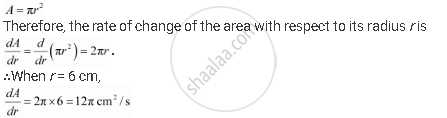Share

# The Rate of Change of the Area of a Circle with Respect to Its Radius R At R = 6 Cm is - CBSE (Commerce) Class 12 - Mathematics

ConceptRate of Change of Bodies Or Quantities

#### Question

The rate of change of the area of a circle with respect to its radius r at r = 6 cm is

(A) 10π

(B) 12π

(C) 8π

(D) 11π

#### Solution

The area of a circle (A) with radius (r) is given by,Hence, the required rate of change of the area of a circle is 12π cm2/s.